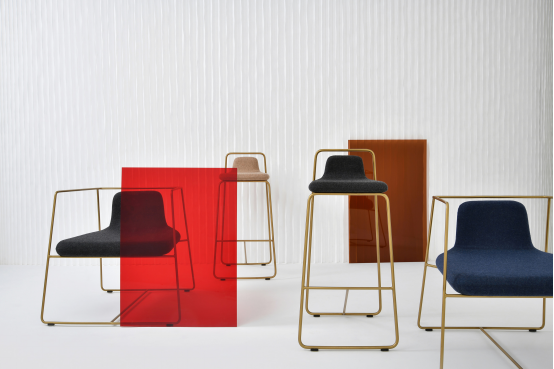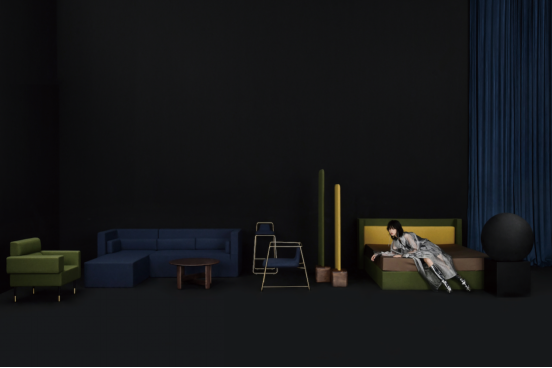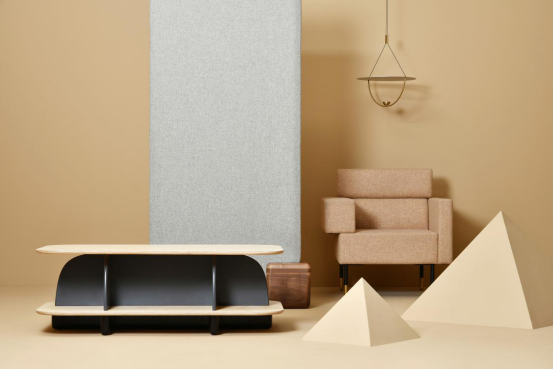|

# 梵几合作设计师周宸宸:设计是承载生活情感的雕塑

作为国内的独立设计师家具品牌，梵几始终保持其对家具设计及品质真实性的高度追求。2018年4月，梵几历经一年多筹备的实验性支线FF系列正式上市。此次，梵几首邀国内设计师周宸宸Frank Chou作为合作设计师。在他的设计下，FF系列融合了独特的当代设计语言，现代简约而不失温度感。搜狐焦点家居专访设计师周宸宸，了解他对于家居设计的理解。以下为采访实录。

【搜狐焦点家居】：您做设计的时候最大的灵感来源于哪里呢？

【周宸宸】：其实，我们对“灵感”的理解跟传统意义上的“灵感”或其他设计师对“灵感”的理解不太相似。我们认为灵感是一个广义的概念，涵盖很多内容。灵感可以是一天24小时里所有发生过的事情，可以是一句话，可以是一件事，可以是突如其来闪过脑海的一个想法。灵感来自生活各方面的信息，所有的信息都能激发你产生新的思考、新的理解。我常认为，比灵感更重要的，是捕捉信息、捕捉闪光点，并把这些零碎的东西转化整合的能力。【搜狐焦点家居】：大家对于你的关注度不仅仅在于在国内的影响力，还有国际上的影响力。在国际舞台上，您想呈现怎样的中国家居设计？

【周宸宸】：我觉得，呈现出来的具体的家居设计是可遇不可求的，具体的设计模样可能并不会是由我们的想象决定。我们希望，在国际舞台上呈现的中国家居设计能符合基本的水准，就是在大众认可的及格线之上。其次，呈现出来的中国家居设计能够表达中国设计、代表中国设计风格。比方说，当一个外国参观者看到并了解某件设计作品之后，他能够体会到隐含在这个作品中的中国设计内涵。

【搜狐焦点家居】：去年我们和周小杰老师聊过一个话题，既然所有东西都是一样，为什么我们还要再去设计一把椅子？我想听听您的想法。

【周宸宸】：在我看来，人们难免有“喜新厌旧”的习惯。每一把椅子，它的物理性能和实用功能早就达成。但是，人们还是希望椅子能有变化，能看到不一样的东西。你拥有了一把世界上最经典的椅子，但也许10年之后，你会觉得这是一把无趣的椅子，同时你想拥有一把新的、不一样的椅子。从另外一个角度看，我觉得设计就像生活的雕塑，承载了人们的情感寄语。每一首歌，每一篇文章承载的不仅是单一的视觉上或者听觉上的东西，而且还承载着作者想要传递的感情。一把椅子也是如此，从物理层面来看，它早就满足了使用属性。但从情感层面来看，它还有非常多的空白等待着设计师在整个的社会中寻找精神的共鸣体。FF系列形象片

【搜狐焦点家居】：所以，人们需要精神上的共鸣体，所以才需要设计新的东西吗？

【周宸宸】：我觉得，家居设计产品是所有设计产品中非常特殊的一个品类。首先，家居产品能在人们的生活存在一段很长的时间。其次，家居设计是设计思维的一个载体，也许它承载着一个时代变化，人们对设计的理解。狭义上，中国家居设计是中国现代化进程的一个缩影。

【搜狐焦点家居】：人们常说，好的设计师一定不是一个好的商人。设计跟艺术一样，需要更开阔的思维，而商人意味着要切合商业化进程的东西。您是否赞同这句话呢？

【周宸宸】：我并不完全赞同这句话。首先，艺术跟设计的区别非常之大。一个真正合格的设计师，最基础的应该是一个商人。一个产品承载了太多的商业风险和商业诉求，如果这个商品没有办法实现商业价值，它不可能存在于这个市场中，更不可能展现更多价值。所以一个合格的设计师必须要考虑商业化的因素，而艺术家可以完全不用去考虑商业。一个只能够停留在展览馆和美术馆里的设计品并不是产品。FF系列

【搜狐焦点家居】：在您看来，有一些在博物馆展出的物品属于艺术品范畴吗？

【周宸宸】：可以这么理解。这些物品并不是通过产品的方式去影响社会，而是通过艺术传播的方式去影响社会。从这个角度看，这就不是一个严格意义上的产品了。所以，如果一个产品设计师不了解成本控制、商业规律，以及产业规律的话，他可能不是一个合格的设计师。

【搜狐焦点家居】：您会怎么选择价值观相同、很适合在一起合作的对象呢？

【周宸宸】：在选择合作方这件事情上，我们并不会简单地从商业利益方面考虑。我们希望，合作方有对“设计”有一个比较客观以及深刻的理解。这个是合作的基础。其次，双方在行业的社会责任方面的看法是一致的，或者是契合的。这是我们选择合作方的标准。

【搜狐焦点家居】：感谢周老师接受我们的采访！

`声明：本文由入驻焦点开放平台的作者撰写，除焦点官方账号外，观点仅代表作者本人，不代表焦点立场错误信息举报电话： 400-099-0099，邮箱：jubao@vip.sohu.com，或点此进行意见反馈，或点此进行举报投诉。`A B C D E F G H J K L M N P Q R S T W X Y Z
A - B - C - D - E
• A
• 鞍山
• 安庆
• 安阳
• 安顺
• 安康
• 澳门
• B
• 北京
• 保定
• 包头
• 巴彦淖尔
• 本溪
• 蚌埠
• 亳州
• 滨州
• 北海
• 百色
• 巴中
• 毕节
• 保山
• 宝鸡
• 白银
• 巴州
• C
• 承德
• 沧州
• 长治
• 赤峰
• 朝阳
• 长春
• 常州
• 滁州
• 池州
• 长沙
• 常德
• 郴州
• 潮州
• 崇左
• 重庆
• 成都
• 楚雄
• 昌都
• 慈溪
• 常熟
• D
• 大同
• 大连
• 丹东
• 大庆
• 东营
• 德州
• 东莞
• 德阳
• 达州
• 大理
• 德宏
• 定西
• 儋州
• 东平
• E
• 鄂尔多斯
• 鄂州
• 恩施
F - G - H - I - J
• F
• 抚顺
• 阜新
• 阜阳
• 福州
• 抚州
• 佛山
• 防城港
• G
• 赣州
• 广州
• 桂林
• 贵港
• 广元
• 广安
• 贵阳
• 固原
• H
• 邯郸
• 衡水
• 呼和浩特
• 呼伦贝尔
• 葫芦岛
• 哈尔滨
• 黑河
• 淮安
• 杭州
• 湖州
• 合肥
• 淮南
• 淮北
• 黄山
• 菏泽
• 鹤壁
• 黄石
• 黄冈
• 衡阳
• 怀化
• 惠州
• 河源
• 贺州
• 河池
• 海口
• 红河
• 汉中
• 海东
• 怀来
• I
• J
• 晋中
• 锦州
• 吉林
• 鸡西
• 佳木斯
• 嘉兴
• 金华
• 景德镇
• 九江
• 吉安
• 济南
• 济宁
• 焦作
• 荆门
• 荆州
• 江门
• 揭阳
• 金昌
• 酒泉
• 嘉峪关
K - L - M - N - P
• K
• 开封
• 昆明
• 昆山
• L
• 廊坊
• 临汾
• 辽阳
• 连云港
• 丽水
• 六安
• 龙岩
• 莱芜
• 临沂
• 聊城
• 洛阳
• 漯河
• 娄底
• 柳州
• 来宾
• 泸州
• 乐山
• 六盘水
• 丽江
• 临沧
• 拉萨
• 林芝
• 兰州
• 陇南
• M
• 牡丹江
• 马鞍山
• 茂名
• 梅州
• 绵阳
• 眉山
• N
• 南京
• 南通
• 宁波
• 南平
• 宁德
• 南昌
• 南阳
• 南宁
• 内江
• 南充
• P
• 盘锦
• 莆田
• 平顶山
• 濮阳
• 攀枝花
• 普洱
• 平凉
Q - R - S - T - W
• Q
• 秦皇岛
• 齐齐哈尔
• 衢州
• 泉州
• 青岛
• 清远
• 钦州
• 黔南
• 曲靖
• 庆阳
• R
• 日照
• 日喀则
• S
• 石家庄
• 沈阳
• 双鸭山
• 绥化
• 上海
• 苏州
• 宿迁
• 绍兴
• 宿州
• 三明
• 上饶
• 三门峡
• 商丘
• 十堰
• 随州
• 邵阳
• 韶关
• 深圳
• 汕头
• 汕尾
• 三亚
• 三沙
• 遂宁
• 山南
• 商洛
• 石嘴山
• T
• 天津
• 唐山
• 太原
• 通辽
• 铁岭
• 泰州
• 台州
• 铜陵
• 泰安
• 铜仁
• 铜川
• 天水
• 天门
• W
• 乌海
• 乌兰察布
• 无锡
• 温州
• 芜湖
• 潍坊
• 威海
• 武汉
• 梧州
• 渭南
• 武威
• 吴忠
• 乌鲁木齐
X - Y - Z
• X
• 邢台
• 徐州
• 宣城
• 厦门
• 新乡
• 许昌
• 信阳
• 襄阳
• 孝感
• 咸宁
• 湘潭
• 湘西
• 西双版纳
• 西安
• 咸阳
• 西宁
• 仙桃
• 西昌
• Y
• 运城
• 营口
• 盐城
• 扬州
• 鹰潭
• 宜春
• 烟台
• 宜昌
• 岳阳
• 益阳
• 永州
• 阳江
• 云浮
• 玉林
• 宜宾
• 雅安
• 玉溪
• 延安
• 榆林
• 银川
• Z
• 张家口
• 镇江
• 舟山
• 漳州
• 淄博
• 枣庄
• 郑州
• 周口
• 驻马店
• 株洲
• 张家界
• 珠海
• 湛江
• 肇庆
• 中山
• 自贡
• 资阳
• 遵义
• 昭通
• 张掖
• 中卫

1室1厅1厨1卫1阳台

1
2
3
4
5

0
1
2

1

1

0
1
2
3报名成功，资料已提交审核A B C D E F G H J K L M N P Q R S T W X Y Z
A - B - C - D - E
• A
• 鞍山
• 安庆
• 安阳
• 安顺
• 安康
• 澳门
• B
• 北京
• 保定
• 包头
• 巴彦淖尔
• 本溪
• 蚌埠
• 亳州
• 滨州
• 北海
• 百色
• 巴中
• 毕节
• 保山
• 宝鸡
• 白银
• 巴州
• C
• 承德
• 沧州
• 长治
• 赤峰
• 朝阳
• 长春
• 常州
• 滁州
• 池州
• 长沙
• 常德
• 郴州
• 潮州
• 崇左
• 重庆
• 成都
• 楚雄
• 昌都
• 慈溪
• 常熟
• D
• 大同
• 大连
• 丹东
• 大庆
• 东营
• 德州
• 东莞
• 德阳
• 达州
• 大理
• 德宏
• 定西
• 儋州
• 东平
• E
• 鄂尔多斯
• 鄂州
• 恩施
F - G - H - I - J
• F
• 抚顺
• 阜新
• 阜阳
• 福州
• 抚州
• 佛山
• 防城港
• G
• 赣州
• 广州
• 桂林
• 贵港
• 广元
• 广安
• 贵阳
• 固原
• H
• 邯郸
• 衡水
• 呼和浩特
• 呼伦贝尔
• 葫芦岛
• 哈尔滨
• 黑河
• 淮安
• 杭州
• 湖州
• 合肥
• 淮南
• 淮北
• 黄山
• 菏泽
• 鹤壁
• 黄石
• 黄冈
• 衡阳
• 怀化
• 惠州
• 河源
• 贺州
• 河池
• 海口
• 红河
• 汉中
• 海东
• 怀来
• I
• J
• 晋中
• 锦州
• 吉林
• 鸡西
• 佳木斯
• 嘉兴
• 金华
• 景德镇
• 九江
• 吉安
• 济南
• 济宁
• 焦作
• 荆门
• 荆州
• 江门
• 揭阳
• 金昌
• 酒泉
• 嘉峪关
K - L - M - N - P
• K
• 开封
• 昆明
• 昆山
• L
• 廊坊
• 临汾
• 辽阳
• 连云港
• 丽水
• 六安
• 龙岩
• 莱芜
• 临沂
• 聊城
• 洛阳
• 漯河
• 娄底
• 柳州
• 来宾
• 泸州
• 乐山
• 六盘水
• 丽江
• 临沧
• 拉萨
• 林芝
• 兰州
• 陇南
• M
• 牡丹江
• 马鞍山
• 茂名
• 梅州
• 绵阳
• 眉山
• N
• 南京
• 南通
• 宁波
• 南平
• 宁德
• 南昌
• 南阳
• 南宁
• 内江
• 南充
• P
• 盘锦
• 莆田
• 平顶山
• 濮阳
• 攀枝花
• 普洱
• 平凉
Q - R - S - T - W
• Q
• 秦皇岛
• 齐齐哈尔
• 衢州
• 泉州
• 青岛
• 清远
• 钦州
• 黔南
• 曲靖
• 庆阳
• R
• 日照
• 日喀则
• S
• 石家庄
• 沈阳
• 双鸭山
• 绥化
• 上海
• 苏州
• 宿迁
• 绍兴
• 宿州
• 三明
• 上饶
• 三门峡
• 商丘
• 十堰
• 随州
• 邵阳
• 韶关
• 深圳
• 汕头
• 汕尾
• 三亚
• 三沙
• 遂宁
• 山南
• 商洛
• 石嘴山
• T
• 天津
• 唐山
• 太原
• 通辽
• 铁岭
• 泰州
• 台州
• 铜陵
• 泰安
• 铜仁
• 铜川
• 天水
• 天门
• W
• 乌海
• 乌兰察布
• 无锡
• 温州
• 芜湖
• 潍坊
• 威海
• 武汉
• 梧州
• 渭南
• 武威
• 吴忠
• 乌鲁木齐
X - Y - Z
• X
• 邢台
• 徐州
• 宣城
• 厦门
• 新乡
• 许昌
• 信阳
• 襄阳
• 孝感
• 咸宁
• 湘潭
• 湘西
• 西双版纳
• 西安
• 咸阳
• 西宁
• 仙桃
• 西昌
• Y
• 运城
• 营口
• 盐城
• 扬州
• 鹰潭
• 宜春
• 烟台
• 宜昌
• 岳阳
• 益阳
• 永州
• 阳江
• 云浮
• 玉林
• 宜宾
• 雅安
• 玉溪
• 延安
• 榆林
• 银川
• Z
• 张家口
• 镇江
• 舟山
• 漳州
• 淄博
• 枣庄
• 郑州
• 周口
• 驻马店
• 株洲
• 张家界
• 珠海
• 湛江
• 肇庆
• 中山
• 自贡
• 资阳
• 遵义
• 昭通
• 张掖
• 中卫• 手机• 分享
• 设计
免费设计
• 计算器
装修计算器
• 入驻
合作入驻
• 联系
联系我们
• 置顶
返回顶部